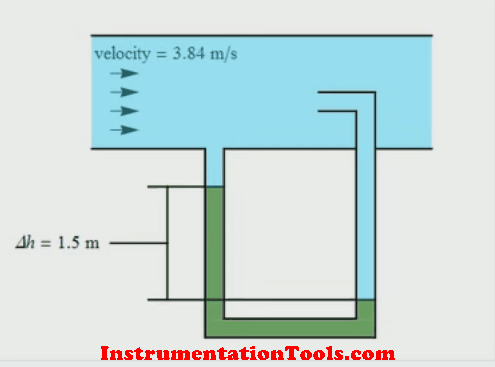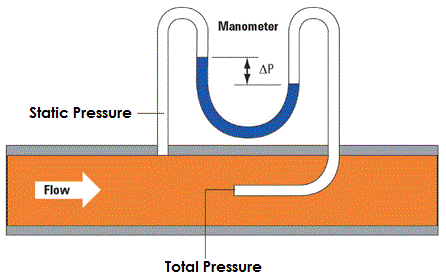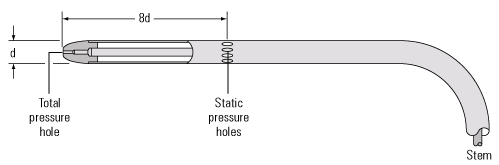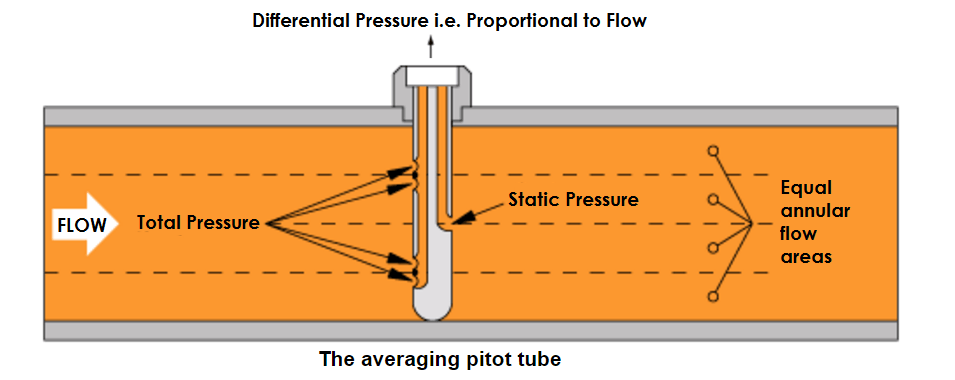# Pitot Tube Working Principle

The Pitot tube is named after Henri Pitot who used a bent glass tube to measure velocities in a river in France in the 1700s. Pitot tubes can be very simple devices with no moving parts used to measure flow velocities.

Pitot tubes are a common type of insertion flowmeter. The below animation shows the basics for a Pitot tube, where a pressure is generated in a tube facing the flow, by the velocity of the fluid.

This ‘velocity’ pressure is compared against the reference pressure (or static pressure) in the pipe, and the velocity can be determined by applying a simple equation.

## Pitot TubeNote: In Animation from right to left: The tube inserted in the center of the pipe is used to measure Total Pressure and the next second tube is used to measure the static pressure.

When the flow rate through the pipe changes, the pressures at the total pressure tube and static pressure tube varies with respect to the flow velocities. The difference between the total pressure and static pressure is used to measure the proportional flow rate passing through the pipe.

A DP type transmitter is used to measure the difference between total pressure and static pressure and it is converted into proportional flow rate.In practice, two tubes inserted into a pipe would be cumbersome, and a simple Pitot tube will consist of one unit as shown in Below Figure. Here, the hole measuring the velocity pressure and the holes measuring the reference or static pressure are incorporated in the same device.Because the simple Pitot tube (Above Figure ) only samples a single point, and, because the flow profile of the fluid (and hence velocity profile) varies across the pipe, accurate placement of the nozzle is critical. To avoid this type of problems we are using averaging pitot tubes.

Note that a square root relationship exists between velocity and pressure drop (see Below Equation). This limits the accuracy to a small turndown range.Where:

 u1 = The fluid velocity in the pipe Δp = Dynamic pressure – Static pressure ρ = Density

#### There are some practical limitations to the use of a pitot-static tube:

1. If the velocity is low, the difference in pressures is very small and hard to accurately measure with the transducer. Errors in the instrument could be greater than the measurement! So pitot-static tubes don’t work very well for very low velocities.
2. If the velocity is very high (supersonic), we’ve violated the assumptions of Bernoulli’s equation and the measurement is wrong again. At the front of the tube, a shock wave appears that will change the total pressure. There are corrections for the shock wave that can be applied to allow us to use pitot-static tubes for high speed aircraft.
3. If the tubes become clogged or pinched, the resulting pressures at the transducer are not the total and static pressures of the external flow. The transducer output is then used to calculate a velocity that is not the actual velocity of the flow.

## Averaging Pitot tube

The averaging Pitot tube (Beow Figure) was developed with a number of upstream sensing tubes to overcome the problems associated with correctly siting the simple type of Pitot tube.

These sensing tubes sense various velocity pressures across the pipe, which are then averaged within the tube assembly to give a representative flowrate of the whole cross section.### Advantages of the Pitot tube

• Presents little resistance to flow.
• Inexpensive to buy.
• Simple types can be used on different diameter pipes.

### Disadvantages of the Pitot tube:

• Turndown is limited to approximately 4:1 by the square root relationship between pressure and velocity.
• If steam is wet, the bottom holes can become effectively blocked. To counter this, some models can be installed horizontally.
• Sensitive to changes in turbulence and needs careful installation and maintenance.
• The low pressure drop measured by the unit, increases uncertainty, especially on steam.
• Placement inside the pipework is critical.

### Applications for the Pitot tube:

• Occasional use to provide an indication of flow rate
• Determining the range over which a more appropriate steam flow meter may be used.

Reference : spiraxsarco

#### Articles You May Like :

Coriolis Meter Construction

Optical flow meter Principle

Doppler Ultrasonic Meter

Turbine Flow Meter Theory

What is Thermal Mass Meter

Don't Miss Our Updates
Be the first to get exclusive content straight to your email.
We promise not to spam you. You can unsubscribe at any time.
1.2.